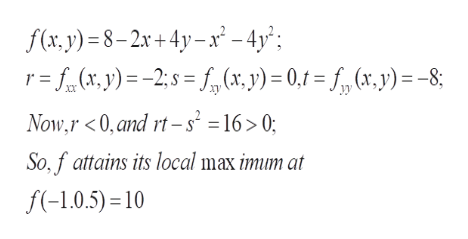# Find the local maximum and minimum values and saddle point(s) of the function. If you have three-dimensional graphing software, graph the function with a domain and viewpoint that reveal all the important aspects of the function. (Enter your answers as a comma-separated list. If an answer does not exist, enter DNE.)f(x, y) = 8 − 2x + 4y − x2 − 4y2

Question
1019 views

Find the local maximum and minimum values and saddle point(s) of the function. If you have three-dimensional graphing software, graph the function with a domain and viewpoint that reveal all the important aspects of the function. (Enter your answers as a comma-separated list. If an answer does not exist, enter DNE.)

f(x, y) = 8 − 2x + 4y − x2 − 4y2
check_circle

Step 1

To analyze the given function for and at the critical points

Step 2

The critical points of the given function of x and y are obtained by settting the partial derivatives to be 0. we find that the only critical point is at (x,y) = (-1, 0.5)

Step 3

To analyze further , consider the second partial derivatives. From the general theory, it follows that ...help_outlineImage Transcriptionclosef(x,y) 8-2x+4y-x-4y'; r=fy)2= (x, y) = 0,1 = fg (x,y)=-8; Now,r <0, and rt-s =16 > 0; So,f attains its local max imum at f(-1.0.5) 10 fullscreen

### Want to see the full answer?

See Solution

#### Want to see this answer and more?

Solutions are written by subject experts who are available 24/7. Questions are typically answered within 1 hour.*

See Solution
*Response times may vary by subject and question.
Tagged in

### Math